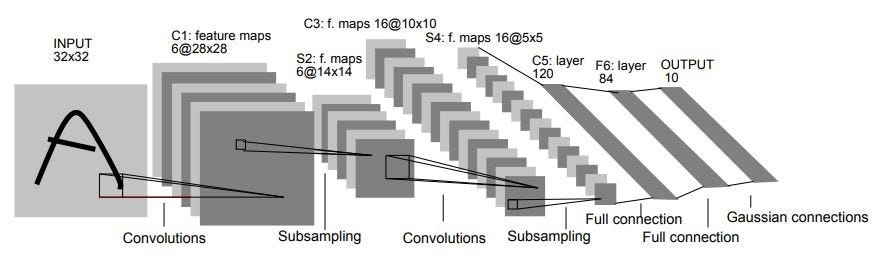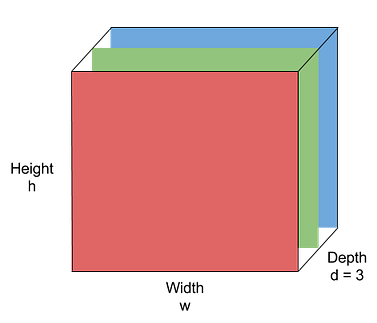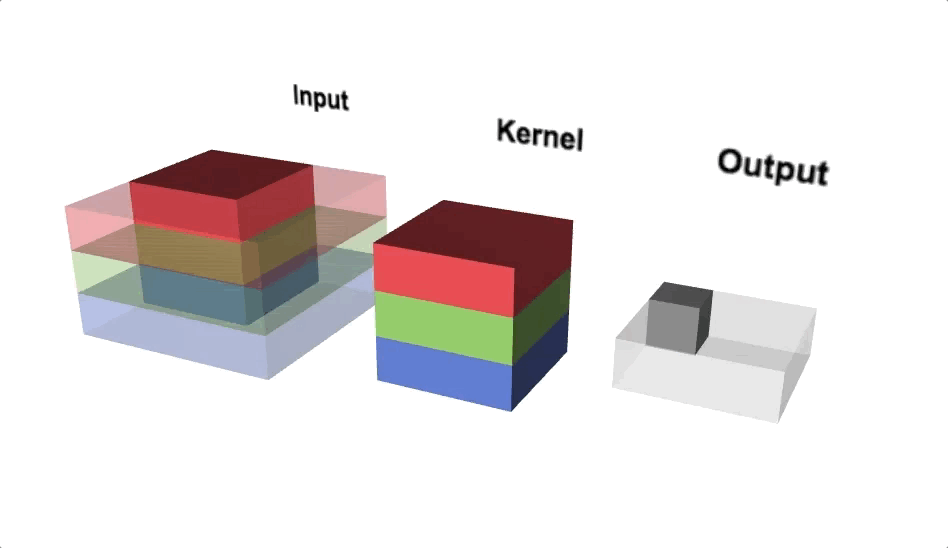1596034380

# TensorFlow vs PyTorch — Convolutional Neural Networks (CNN)

In my previous article, I had given the implementation of a Simple Linear Regression in both TensorFlow and PyTorch frameworks and compared their results. In this article, we shall go through the application of a Convolutional Neural Network (CNN) on a very famous Fashion MNIST dataset using both the frameworks and compare the results.

# Origin —

Let us get a brief idea on both the frameworks and their history. Firstly, PyTorch is an open source machine learning library based on the Torch library. PyTorch was primarily developed by _Facebook’_s AI Research lab (FAIR). It is free and open-source software.

On the other hand, TensorFlow was developed by the Google Brain team for internal Google research purpose. It is widely used for machine learning applications such as neural networks. It is also free and open-source software.

In order to see which framework is more efficient and simpler to use, we shall build a ConvNet using both the frameworks and compare them. For the TensorFlow, we shall be using the _Keras _library.

## Problem —

For comparison of both the frameworks, we will use the famous Fashion-MNIST dataset. It is a datasetof Zalando’s article images consisting of a training set of 60,000 examples and a test set of 10,000 examples. Each example is a 28×28 grayscale image, associated with a label from 10 classes such as shirt, bag, sandal, coat, sneaker etc. We will build a CNN network with a common architecture of LeNet with both the frameworks and compare their results.

# Step 1: Importing the Libraries

In the first step, we will be importing the required libraries for both the TensorFlow and PyTorch frameworks.

``````import numpy as np
import matplotlib.pyplot as plt
%matplotlib inline

#TensorFlow - Importing the Libraries
import tensorflow as tf
from tensorflow import keras

#PyTorch - Importing the Libraries
import torch
import torch.nn as nn
import torchvision
import torchvision.transforms
view raw
ptvstf1.py hosted with ❤ by GitHub
``````

Importing the Libraries

# Step 2: Getting and Splitting the Dataset

In the next step, we will get the Fashion MNIST dataset. As it is a very common dataset, both the frameworks have preloaded functions that enable us to download the dataset and use it.

``````#PyTorch - Getting and Splitting the Dataset
transforms = torchvision.transforms.Compose([torchvision.transforms.ToTensor()])
train_dataset_pytorch = torchvision.datasets.FashionMNIST(root='./data/',
train=True,
transform=transforms,
test_dataset_pytorch = torchvision.datasets.FashionMNIST(root='.data/',
train=False,
transform=transforms,
view raw
ptvstf2.py hosted with ❤ by GitHub
``````

## PyTorch

In PyTorch, the built-in module is used to load the dataset. As we need the data in the form of tensors,the data is converted into tensors by using the`torchvision.transforms.ToTensor()`and assigning it to the variable `transforms. `The data is then downloaded with `torchvision.datasets`. The data is correspondingly split into the train_dataset and the test_dataset.

## TensorFlow

``````#TensorFlow - Getting and Splitting the Dataset
fashion_mnist = keras.datasets.fashion_mnist
(train_images_tf, train_labels_tf), (test_images_tf, test_labels_tf) = fashion_mnist.load_data()
view raw
ptvstf3.py hosted with ❤ by GitHub
``````

On the other hand, getting the data from the keras library using TensorFlow is more simpler compared to the PyTorch version. The dataset is loaded from `keras.datasets` and it is split into the train_images and test_images accordingly.

In this step, we shall load the data into the training set and test set. Additionally, we shall also view how the image is stored in both the frameworks.

## PyTorch

``````#PyTorch - Loading the Data
def imshowPytorch(img):
npimg = img.numpy()
plt.imshow(np.transpose(npimg, (1, 2, 0)))
batch_size=32,
shuffle=False)
batch_size=32,
shuffle=False)

images, label = data_iter.next()
imshowPytorch(torchvision.utils.make_grid(images))
print(label)
view raw
ptvstf4.py hosted with ❤ by GitHub
``````

In PyTorch, the images are stored in the ranges 0–1 and hence the images are displayed in B/W. The images are loaded into the train_loader and the test_loader using the `DataLoader`function. Additionally, the label of the image is also printed.

#towards-data-science #mls #artificial-intelligence #machine-learning-ai #machine-learning #deep learning

## Buddha Community1598298420

## Coding Convolutional Neural Networks (CNN) with TensorFlow

_CNNs _are one of the state of the art, Artificial Neural Network design architecture, with one of the best deep learning tools in areas such as image recognition and classification. The Basic Principle behind the working of CNN is the idea of Convolution, producing filtered Feature Maps stacked over each other.

We’ll be using MNIST dataset which is readily available in different libraries. We’ll be importing our dataset from tensorflow library.

Code has been written in a generic template so as to do very minimal modifications and can run on many datasets with very little change.

Every CNN is made up of multiple layers, the three main types of layers are convolutional, pooling, and fully-connected.

1. Convolution Layer (Conv Layer)

The Conv layer is the core building block of a Convolutional Neural Network. The primary purpose of Conv layer is to extract features from the input image.

The convolutional layer can be thought of as the feature extractor of this network, it learns to find spatial features in an input image. This layer is produced by applying a series of many different image filters, also known as convolutional kernels, to an input image. These filters are very small grids of values that slide over an image, pixel-by-pixel, and produce a filtered output image that will be about the same size as the input image. Multiple kernels will produce multiple filtered, output images.

2. Pooling Layer

Pooling layer reduces the size of feature maps by using some functions to summarize sub-regions, such as taking the average or the maximum value. Pooling works by sliding a window across the input and feeding the content of the window to a pooling function.

3. Fully Connected Layer

The Fully Connected layer is configured exactly the way its name implies: it is fully connected with the output of the previous layer. A fully connected layer takes all neurons in the previous layer (be it fully connected, pooling, or convolutional) and connects it to every single neuron it has.

#data-science #deep-learning #neural-networks #tensorflow #cnn1623135499

## No Code introduction to Neural Networks

### The simple architecture explained

Neural networks have been around for a long time, being developed in the 1960s as a way to simulate neural activity for the development of artificial intelligence systems. However, since then they have developed into a useful analytical tool often used in replace of, or in conjunction with, standard statistical models such as regression or classification as they can be used to predict or more a specific output. The main difference, and advantage, in this regard is that neural networks make no initial assumptions as to the form of the relationship or distribution that underlies the data, meaning they can be more flexible and capture non-standard and non-linear relationships between input and output variables, making them incredibly valuable in todays data rich environment.

In this sense, their use has took over the past decade or so, with the fall in costs and increase in ability of general computing power, the rise of large datasets allowing these models to be trained, and the development of frameworks such as TensforFlow and Keras that have allowed people with sufficient hardware (in some cases this is no longer even an requirement through cloud computing), the correct data and an understanding of a given coding language to implement them. This article therefore seeks to be provide a no code introduction to their architecture and how they work so that their implementation and benefits can be better understood.

Firstly, the way these models work is that there is an input layer, one or more hidden layers and an output layer, each of which are connected by layers of synaptic weights¹. The input layer (X) is used to take in scaled values of the input, usually within a standardised range of 0–1. The hidden layers (Z) are then used to define the relationship between the input and output using weights and activation functions. The output layer (Y) then transforms the results from the hidden layers into the predicted values, often also scaled to be within 0–1. The synaptic weights (W) connecting these layers are used in model training to determine the weights assigned to each input and prediction in order to get the best model fit. Visually, this is represented as:

#machine-learning #python #neural-networks #tensorflow #neural-network-algorithm #no code introduction to neural networks1594935240

## Convolutional Neural Networks(CNN’s) — A practical perspective

Hello everyone,

Hoping that everyone is safe and doing well. In this blog, we will look into some concepts of CNN’s for image classification that are often missed or misunderstood by beginners (including me till some time back). This blog requires the reader to have some basic idea of how CNN’s work. However, we will cover the important aspects of CNN’s before getting deeper into advanced topics.

After this, we will look at a machine learning technique called Transfer learning and how it is useful in training a model with less data on a deep learning framework. We will train an image classification model on top of Resnet34 architecture using the data that contains digitally recorded heartbeats of human beings in the form of audio (.wav) files. In the process, we will convert each of these audio files into an image by converting them to spectrograms using a popular python audio library called Librosa. In the end, we will examine the model with popular error metrics and check its performance.

## CNN’s for image classification:

Neural networks with more than 1 convolution operations are called convolutional neural networks (CNN). Input of a CNN contains images with numerical values in each pixel arranged spatially along the width, height and depth (channels). The goal of the total architecture is to get a probability score of an image belonging to a certain class by learning from these numerical values arranged spatially. In the process, we perform operations like pooling and convolutions on these numerical values to squeeze and stretch them along the depth.

An image typically contains three layers namely RGB (Red, Green, Blue).Image by Purit Punyawiwat, Source : Datawow

## Main operations in CNN’s

Convolution operation

Convolution operation is (w.x+b) applied to all the different spatial localities in the input volume. Using more number of convolution operations helps to learn a particular shape even if its location in the image is changed.

Example: Generally clouds are present on the top of a landscape image. If an inverted image is fed into a CNN, more number of convolutional operations makes sure that the model identifies the cloud portion even if it is inverted.

Mathematical Expression: x_new = w.x + b where w is the filter/kernel, b is the bias and x is part of a hidden layer output. Both w and  _b _are different for every convolution operation applied on different hidden layers.Convolution Operations(Source: Lecture 22-EECS251, inst.eecs.berkley.edu)

Pooling

Pooling reduces the spatial dimensions of each activation map (output after convolution operation) while aggregating the localised spatial information. Pooling helps to squeeze the output from hidden layers along height and width. If we consider maximum value within the non-overlapping sub-regions then it is called Max-pooling. Max-pooling also adds non-linearity to the model.

#pytorch #deep-learning #transfer-learning #audio-classification #convolutional-network #neural networks1600797360

## Fashion MNIST

Fashion Mnist is a Dataset created by Zolando Fashion Wear to replace the Original Mnist and at the same time increasing the difficulty.

This blog post is all about how to create a model to predict fashion mnist images and shows how to implement convolutional layers in the network

Let’s look at the code

#convolutional-neural-net #pytorch #convolutional-network #deep-learning1602983880

## Convolutional Neural Networks (CNN) Model from scratch for Object Detection

If you can’t explain it simply, you don’t understand it well enough - Einstein, the Man and His Achievement By G. J. Whitrow, Dover Press 1973.

CNN Model made from scratch, using the most popular Kaggle dataset  Fruits-360 and obtaining 98% accuracy.

Step 1- Importing Dataset From Kaggle to Google Colab

``````!pip install -q kaggle
``````! mkdir ~/.kaggle